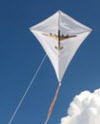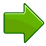# Kite

A Kite is a flat shape with straight sides.
It has two pairs of equal-length adjacent (next to each other) sides.It often looks like a kite!Two pairs of sidesEach pair is two equal-length sides that are adjacent (they meet)The angles are equal where the two pairs meetDiagonals (dashed lines) cross at right angles, and one of the diagonals bisects (cuts equally in half) the other

Play with a Kite:

## Area of a Kite

#### Method 1:Multiply the lengths of the diagonals and then divide by 2 to find the Area: Area = p × q2

### Example: A kite has diagonals of 3 cm and 5 cm, what is its Area?

Area = 3 cm × 5 cm2 = 7.5 cm2

#### Method 2:Multiply the lengths of two unequal sides by the sine of the angle between them: Area = a × b × sin(C)### Example: You don't want to get wet measuring the diagonals of a kite-shaped swimming pool. So you measure unequal side lengths of 5.0 m and 6.5 m with an angle between them of 60°. What is its Area?

 Area = a × b × sin(C) = 5.0 × 6.5 × sin(60°) = 5.0 × 6.5 × 0.866... = 28.1 m2 (to 1 decimal)

#### Method 3:

If you can draw your Kite, try the Area of Polygon by Drawing tool.

## Perimeter of a Kite

The Perimeter is the distance around the edges.The Perimeter is 2 times (side length a + side length b): Perimeter = 2(a + b)

### Example: A kite has side lengths of 12 m and 10m, what is its Perimeter?

Perimeter = 2 × (12 m + 10 m) = 2 × 22 m = 44 m

## Rhombus and Square

When all sides have equal length the Kite will also be a Rhombus.

When all the angles are also 90° the Kite will be a Square.

A Square is a Kite? Yes! So it doesn't always look like the kite you fly.A Dart

## Dart

A concave Kite is called a Dart

9141, 9142, 9143, 9144, 9145, 9146, 9147, 9148, 9149, 9150